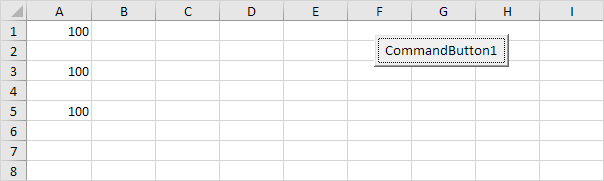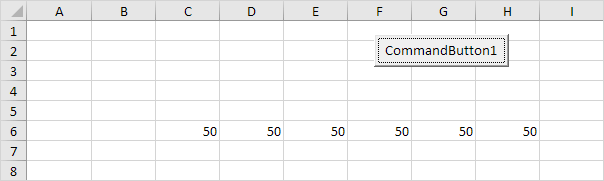# Step Keyword

You can use the Step keyword in Excel VBA to specify a different increment for the counter variable of a loop.

1. Place a command button on your worksheet and add the following code lines:

Dim i As Integer

For i = 1 To 6 Step 2
Cells(i, 1).Value = 100
Next i

Result when you click the command button on the sheet:Explanation: The code lines between For and Next will be executed three times. For i = 1, Excel VBA enters the value 100 into the cell at the intersection of row 1 and column 1. When Excel VBA reaches Next i, it increases i with 2 and jumps back to the For statement. For i = 3, Excel VBA enters the value 100 into the cell at the intersection of row 3 and column 1, etc.

2. Place a command button on your worksheet and add the following code lines:

Dim j As Integer

For j = 8 To 3 Step -1
Cells(6, j).Value = 50
Next j

Result when you click the command button on the sheet:Explanation: The code lines between For and Next will be executed six times. For j = 8, Excel VBA enters the value 50 into the cell at the intersection of row 6 and column 8. When Excel VBA reaches Next j, it decreases j with 1 and jumps back to the For statement. For j = 7, Excel VBA enters the value 50 into the cell at the intersection of row 6 and column 7, etc.

Go to Next Chapter: Macro Errors